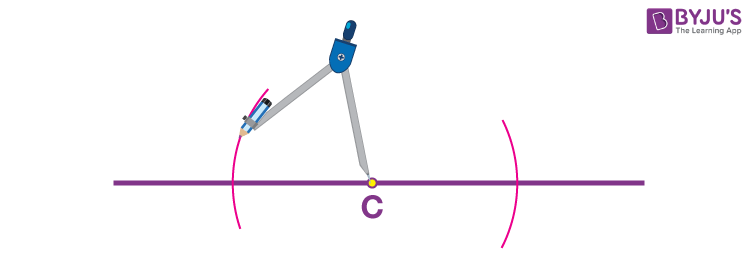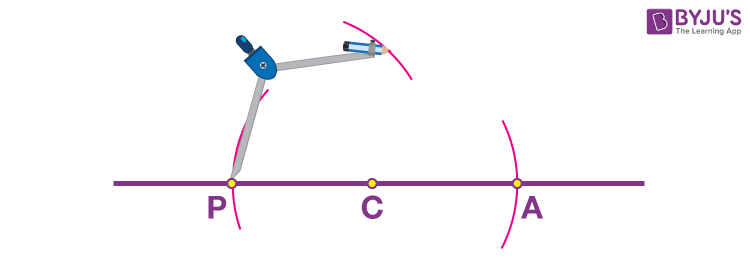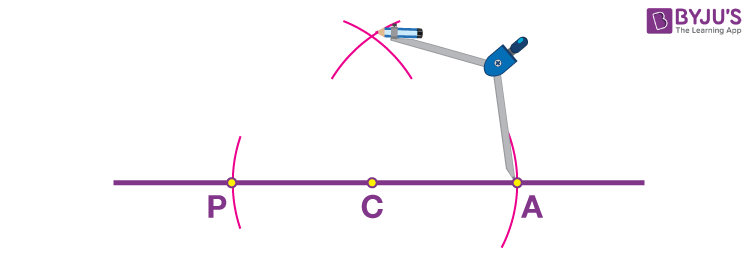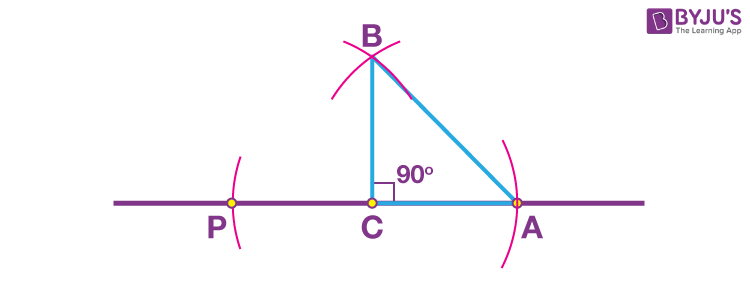# Right Angled Triangle: Constructions (RHS)

Construction of a right-angled triangle is possible when the Hypotenuse and one side from the remaining two sides are known to us. A right-angled triangle is a triangle which has one of its three angles equal to 90 degrees. The other two angles are acute angles. The side opposite the right angle is the longest side and is called the hypotenuse. The side which makes the right angle with the base is called perpendicular.

The requirements for the construction are a ruler and a compass.

## Constructions of Right-angled Triangle

Let us construct a right-angled triangle ABC, right-angled at C. Consider the length of the hypotenuse AB = 5 cm and side CA = 3 cm. The steps for construction are:

• Step 1: Draw a horizontal line of any length and mark a point C on it.• Step 2: Set the compass width to 3 cm.
• Step 3: Place the pointer head of the compass on point C and mark an arc on both sides of C.• Step 4: Mark the points as P and A where the arcs cross the line.
• Step 5: Set the compass width to the length of the hypotenuse, that is, 5 cm.
• Step 6: Place the pointer head of the compass on point P and mark an arc above C.• Step 7: Repeat step 6 from point A.• Step 8: Mark the point as B where the two arcs cross each other.
• Step 9: Join the points B and A as well as B and C with the ruler.Thus, you obtain a right-angled triangle ACB of the required measurements.

### Video Lesson on Types of Triangles## Frequently Asked Questions on Right Angled Triangle Construction

Q1

### Which angle is called the right angle?

An angle which makes 90 degrees is called a right angle.

Q2

### In a right-angle triangle what is the largest side?

The hypotenuse is the largest side of the right-angle triangle

Q3

### In a right-angled triangle, what is the largest angle?

In a right-angled triangle the largest angle is 90 degrees.

Q4

### In a right triangle sum of two sides is greater than the which side?

In a right triangle sum of two sides is greater than the third side.

Q5

### In a right triangle other than the right angle, what is the sum of the other two angles?

In a right triangle the sum of two angles is equal to the right angle.